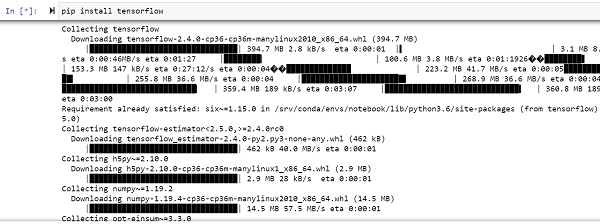# How can TensorFlow used to train a linear model using Python?

PythonServer Side ProgrammingProgramming

Tensorflow is a machine learning framework that is provided by Google. It is an open−source framework used in conjunction with Python to implement algorithms, deep learning applications and much more. It is used in research and for production purposes.

The ‘tensorflow’ package can be installed on Windows using the below line of code −

pip install tensorflow

Tensor is a data structure used in TensorFlow. It helps connect edges in a flow diagram. This flow diagram is known as the ‘Data flow graph’. Tensors are nothing but multidimensional array or a list. They can be identified using three main attributes −

Rank − It tells about the dimensionality of the tensor. It can be understood as the order of the tensor or the number of dimensions in the tensor that has been defined.

Type − It tells about the data type associated with the elements of the Tensor. It can be a one dimensional, two dimensional or n dimensional tensor.

Shape − It is the number of rows and columns together.

We will be using the Jupyter Notebook to run the code. TensorFlow can be installed on Jupyter Notebook using ‘pip install tensorflow’.Following is the code −

## Example

import tensorflow as tf

A = tf.get_variable("A", initializer=tf.constant([0.1]))
b = tf.get_variable("b", initializer=tf.constant([0.0]))

x = tf.placeholder(tf.float32)
y = tf.placeholder(tf.float32)

my_model = A * x + b

lossVal = tf.reduce_sum(tf.square(my_model − y))

train = my_optimizer.minimize(lossVal)

x_train = [1.0, 2.5, 3.8, 4.9]
y_train = [1.7, 3.0, 6.6, 6.8]

with tf.Session() as sess:
sess.run(tf.global_variables_initializer())
for i in range(1000):
sess.run(train, {x:x_train, y:y_train})
if i%100==0:
l_cost = sess.run(lossVal, {x:x_train, y:y_train})
print(f"i: {i} cost: {l_cost}")
l_A, l_b, l_cost = sess.run([A, b, lossVal], {x:x_train, y:y_train})
print(f"A: {l_A} b: {l_b} cost: {l_cost}")

## Output

i: 0 cost: 1.7808341979980469
i: 100 cost: 1.6947696208953857
i: 200 cost: 1.691591501235962
i: 300 cost: 1.6913959980010986
i: 400 cost: 1.6913844347000122
i: 500 cost: 1.6913840770721436
i: 600 cost: 1.6913843154907227
i: 700 cost: 1.691383719444275
i: 800 cost: 1.6913838386535645
i: 900 cost: 1.6913845539093018
A: [1.4599526] b: [0.07214472] cost: 1.6913845539093018

## Explanation

• Import the required packages and provide an alias for it, for ease of use.

• A variable named ‘my_model´ is defined, that stores the general format for a linear model.

• This linear model is trained using the ‘GradientDescentOptimizer’.

• The training is done such that the loss is minimal, which is ensured using ‘minimize’ method.

• Two lists are created that store the training data.

• This data is trained, and the predicted values are displayed on the console.